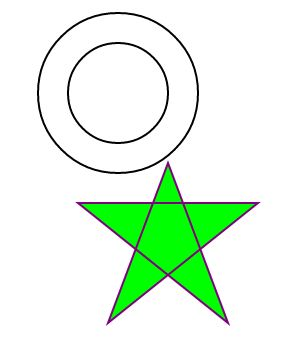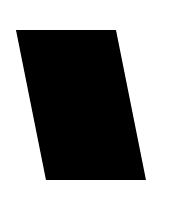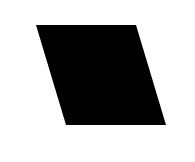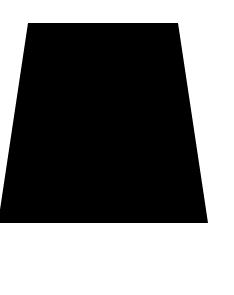HTML 5 TutorialsHow to draw shapes using SVG in HTML5

How to draw shapes using SVG in HTML5

We know SVG or scalable vector graphics is a powerful way to represent graphics in browser. In our blog today we will create various shapes using SVG. Our shapes include Star, Concentric Circle, Rhombus, Parallelogram and Trapezium. Let us start with our coding

Step 1

Create a HTML page and write the following for concentric circles

<!DOCTYPE html PUBLIC "-//W3C//DTD XHTML 1.0 Transitional//EN" "http://www.w3.org/TR/xhtml1/DTD/xhtml1-transitional.dtd">
<html xmlns="http://www.w3.org/1999/xhtml">
//Here I mentioned the name space of the SVG , as the format is xml so it is written as xmlns.

<meta http-equiv="Content-Type" content="text/html; charset=utf-8" />
<title>STAR AND CONCENTRIC CIRCLE</title>

<body><br />
<h2>USE OF SVG TO DRAW TWO CONCENTRIC CIRCLES</h2>
<svg xmlns="http://www.w3.org/2000/svg" version="1.1">
<circle cx="200" cy="100" r="80" stroke="black"
stroke-width="2" fill="white"/>

here is the code above to draw a circle with center coordinate as cx and cy can be defined but if they are not defined they are considered as (0,0). r is the value of the radius. Stroke width is the width of the border. Fill is the color to be filled inside the circle,I take it as white to show concentricity.

<circle cx="200" cy="100" r="50" stroke="black"
stroke-width="2" fill="white"/>

The above is the second circle with bigger radius to make it concentric with the first.

Step 2

We now will write the code to draw a Star.

<polygon points="250,170, 190,330 ,340,210 ,160,210 ,310,330" style="fill:lime;stroke:purple;stroke-width:2;"/>
</svg>

<br />
<h2>USE OF SVG TO DRAW STAR</h2>

In the above code we draw polygon points to draw the shape of the star.

Step 3

To draw a parallelogram we do the following

<svg xmlns="http://www.w3.org/2000/svg" version="1.1" / >
<path d="M50 100 L150 100 L180 250 L80 250 Z" />
</svg>

/M simply means move to the initial point means starting point.
//L simply means to draw a line from initial point to the point defined.
//z is used to draw closed path.
//We can design any figure simply by adjusting the value of coordinates of lines we defined.

Step 4

Draw a Rhombus similar to the following code

<svg xmlns="http://www.w3.org/2000/svg" version="1.1" / >
<path d="M50 100 L150 100 L180 200 L80 200 Z" />
</svg>

we draw a trapezium like the following

<svg xmlns="http://www.w3.org/2000/svg" version="1.1">
<path d="M50 100 L200 100 L230 300 L20 300 Z" />
</svg>

Output

The output will be the followingThe Bitcoin ATMs All Across The World

21,082Fans
4,210Followers
101,000Subscribers